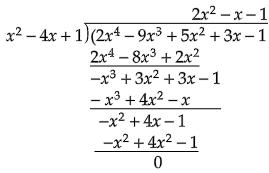# Find all zeroes of the polynomial $( 2x^{4}-9x^{3}+5x^{2}+3x-1)$ if two of its zeroes are $(2+\sqrt{3}) and (2-\sqrt{3)}$.

Given: Polynomial $( 2x^{4}-9x^{3}+5x^{2}+3x-1)$ and two of its zeroes are$( 2+\sqrt{3})$ and $( 2-\sqrt{3})$.

To do: To find all its zeroes.

Solution:

It is given that (2+\sqrt{3}) and (2-\sqrt{3} ) are two zeroes

$[x- (2+\sqrt{3})][x-(2-\sqrt{3} )] =(x- 2-\sqrt{3})(x- 2+\sqrt{3})$

$=[(x-2)-\sqrt{3}][(x-2)-\sqrt{3}]$

$=(x-2)^{2}-(\sqrt{3})^{2}$

$=x^{2}+4-4x-3$

$=x^{2}-4x+1$

$x^{2}-4x+1$ is a factor of the given polynomial.

On dividing the given polynomial by this factor.Hence   $2x^{2}-x-1$ is also a factor of the given polynomial.

$2x^{2}-x-1=2x^{2}-2x+x-1$

$=2x( x-1)+( x-1)$

$=( x-1)( 2x+1)$

if $x-1=0$

$x=1$

If $2x+1=0$

$x=-\frac{1}{2}$

Hence the other two zeroes are 1 and $-\frac{1}{2}$. of the given polynomial.

Updated on: 10-Oct-2022

44 Views